# CRC校验原理探究与算法实现

1. 模二运算
2. 基本原理
3. 算例演示
4. 改进、加速与完善。

## 3. 模二运算

1. 模二加减运算

模二加减运算得到的结果相同, 不考虑借位和进位, 其运算规则如下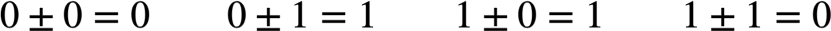2. 模二乘运算

模二乘法即按照模二加法求部分积之和, 不考虑进位.

3. 模二除运算

模二除法即按照模二减法求部分余数, 不考虑借位. 其余所有运算规则和二进制除法相同.

## 4. CRC校验的基本原理

CRC码由有效信息位和校验位组成, 其编码方式如下所述

1. 先将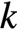位有效信息表达为多项式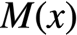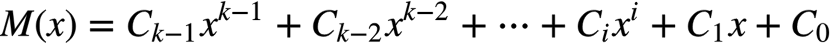上式种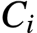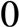或者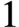.

2.左移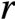位, 可以表示成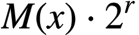. 右边的位用来放置校验位. 其中的取值应满足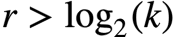.

1. 选择一个生成多项式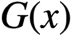, 其最高次幂等于校验位的位数, 最低次幂等于, 即必须是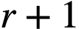位.

2. 用多项式除以生成多项式所得的余数作为校验码.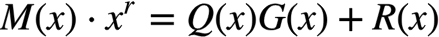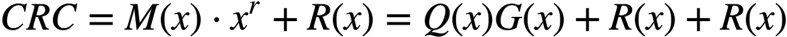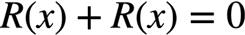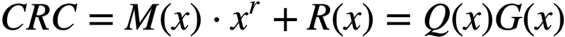## 5. 算例## 6. 改进、加速与完善

### 6. 1 改进位操作问题

1. 首先初始化一个CRC寄存器, 其长度要不小于信息长度和生成多项式长度（实际上生成多项式中因为第一位一定是1所以按照惯例一般省略第一位而且计算长度的时候也不包括第一位， 本例中（也就是前面那个算例）生成多项式为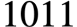,实际上计算中用的是去掉首位的1，为011，而其长度也视为3 ）中最大的那一个. 本例中初始化为1100.

2. 后续的除法操作可以按照下面的方式进行: 如果CRC寄存器中的第一位为1, 则将其左移一位(最后一位补0)后与生成多项式左端对齐后进行异或.(模二加法实际上就是异或运算). 在本例中CRC寄存器第一位是1, 则左移一位后变为1000, 然后与生成多项式011先左端对齐然后异或, 即从而得到更新后的CRC寄存器的值1110.

3. 重复2过程, 重复次数正好为信息长度. 本例中重复四次### 6.2 分段计算

1. 首先计算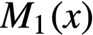的CRC码, 得到余数值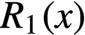.

2. 将余数左移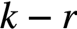位（其中是前面提到的,是生成多项式不计算第一位1的时候的宽度）, 然后加到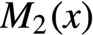上的得到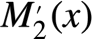.

3.计算CRC码, 得到余数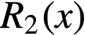.

1. 余数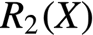即为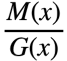的余数, 也就是原信息待求的余数.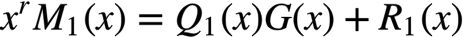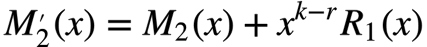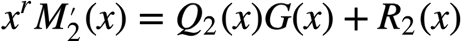### 6.3 查表法加速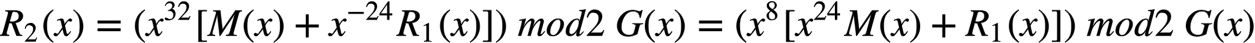的长度也就是CRC寄存器的长度, 记为32bit, 我们把分为两段, 第一段为前8bit, 记为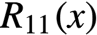, 第二段为后面的24bit, 记为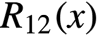, 这样就可以表示为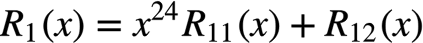## 7. 代码

template<typename PolyType>
class CRC
{
public:
CRC(void)
{
init();
}
CRC(PolyType& initial, PolyType& polynomial, PolyType& xorout, int refin, int refout, size_t _width = sizeof(PolyType)<<3)
{
init();
create(initial, polynomial, xorout, refin, refout, _width);
}
public:
template<typename DataType>
void calculate(DataType& data)
{
BYTE* DataPointer = reinterpret_cast<BYTE*>(&data);
size_t DataSize = sizeof(DataType);
BYTE actualData = 0x00;
for(size_t i = 0; i < DataSize; i++)
{
if(RefIn)
{
actualData = reverseTable[DataPointer[i]];
}
else
{
actualData = DataPointer[i];
}
BYTE index = (CRCreg>>((sizeof(PolyType) - 1)<<3))^actualData;
CRCreg <<= 8;
CRCreg ^= crcTable[index];
}
}
void create(PolyType& initial, PolyType& polynomial, PolyType& xorout, int refin, int refout, size_t _width = sizeof(PolyType)<<3)
{
CRCreg = initial<<((sizeof(PolyType)<<3) - _width);
CRCpoly = polynomial<<((sizeof(PolyType)<<3) - _width);
XORout = xorout<<((sizeof(PolyType)<<3) - _width);
RefIn = refin;
RefOut = refout;
width = _width;
ByteWidth = (width + 7)>>3;
makeTable();
}
void SetCRCreg(PolyType& initial)
{
CRCreg = initial;
}
PolyType GetCRCreg(void)
{
PolyType ret = 0x00;
if(RefOut)
{
BYTE* regPointer = (BYTE*) &CRCreg;
BYTE* retPointer = (BYTE*) &ret;
for(size_t i = 0; i < sizeof(PolyType); i++)
{
retPointer[sizeof(PolyType) - 1 - i] = reverseTable[regPointer[i]];
}
ret <<= sizeof(PolyType) * 8 - width;
}
else
{
ret = CRCreg;
}
ret ^= XORout;
return ret;
}
void reset(void)
{
init();
}
private:
void init(void)
{
RefIn = 0;
RefOut = 0;
width = 0;
ByteWidth = 0;

PolyType one;
one = 0x01;
}
void makeTable(void)
{
for(size_t i = 0; i < 256; i++)
{
BYTE cur = (BYTE) i;
PolyType reg = cur;
reg <<= ((sizeof(PolyType) - 1)<<3);
for(size_t j = 0; j < 8; j++)
{
{
reg <<= 1;
reg ^= CRCpoly;
}
else
{
reg <<= 1;
}
}
crcTable[i] = reg;
}

static BYTE BitMask = {0x01, 0x02, 0x04, 0x08, 0x10, 0x20, 0x40, 0x80};
for(size_t i = 0; i < 256; i++)
{
BYTE cur = (BYTE) i;
BYTE write = 0x00;
for(size_t j = 0; j < 8; j++)
{
{
}
}
reverseTable[i] = write;
}
}
private:
PolyType CRCreg;
PolyType CRCpoly;
PolyType XORout;
int RefIn;
int RefOut;
size_t width;
size_t ByteWidth;
PolyType crcTable;
BYTE reverseTable;
};


## 8. 附件

CRC示例代码：https://static.zmyme.com/code/crc/crcexample.cpp

## 9. 参考资料

1.